# Reference and examples¶

This is the full list of commands available in Shoebot. This documentation is still missing some parts. Refer to the Nodebox documentation for the best reference in the meantime (and where many parts of this documentation are derived from.)

Note that all examples here are drawn on a 100x100 size for simplicity; the Shoebot default is 300x300px.

## Drawing shapes¶

`rect`(x, y, width, height, roundness=0, draw=True, fill=None)

Draw a rectangle.

Parameters
• x – top left x-coordinate

• y – top left y-coordinate

• width – rectangle width

• height – rectangle height

• roundness – rounded corner radius

• draw (boolean) – whether to draw the shape on the canvas or not

• fill – fill color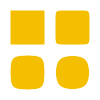```size(200,200)
background(1)
fill(0.95, 0.75, 0)
rect(10, 10, 35, 35)
# see how roundness affects the shape
rect(55, 10, 35, 35, 0.3)
rect(10, 55, 35, 35, 0.7)
rect(55, 55, 35, 35, 1)
```
`ellipse`(x, y, width, height, draw=True)

Draw an ellipse. Same as `oval()`.

Parameters
• x – top left x-coordinate

• y – top left y-coordinate

• width – ellipse width

• height – ellipse height

• draw (boolean) – whether to draw the shape on the canvas or not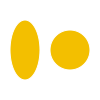```ellipse(10, 20, 30, 60)
ellipse(50, 30, 40, 40) # circle
```
`arrow`(x, y, width, type=NORMAL, draw=True)

Draw an arrow.

Parameters
• x – arrow tip x-coordinate

• y – arrow tip y-coordinate

• width – arrow width (also sets height)

• type (NORMAL or FORTYFIVE) – arrow type

• draw (boolean) – whether to draw the shape on the canvas or not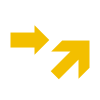```arrow(50, 40, 40) # NORMAL is the default arrow type
arrow(90, 40, 40, FORTYFIVE)
```
`star`(startx, starty, points=20, outer=100, inner=50, draw=True)

Draw a star-like polygon.

Parameters
• startx – center x-coordinate

• starty – center y-coordinate

• points – amount of points

• outer – outer radius

• inner – inner radius

• draw (boolean) – whether to draw the shape on the canvas or not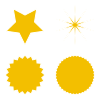```star(25, 25, 5, 20, 10)  # top left
star(75, 25, 10, 20, 3)  # top right
star(25, 75, 20, 20, 17) # bottom left
star(75, 75, 40, 20, 19) # bottom right
```
`line`(x1, y1, x2, y2, draw=True)

Draw a line from (x1,y1) to (x2,y2).

Parameters
• x1 – x-coordinate of the first point

• y1 – y-coordinate of the first point

• x2 – x-coordinate of the second point

• y2 – y-coordinate of the second point

• draw (boolean) – whether to draw the shape on the canvas or not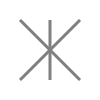```stroke(0.5)
strokewidth(3)
line(20, 20, 80, 80)
line(20, 80, 80, 20)
line(50, 20, 50, 80)
```
`rectmode`(mode=None)

Change the way rectangles are specified. Each mode alters the parameters necessary to draw a rectangle using the `rect()` function.

• use the CORNER mode (default) when you want to specify an origin point and dimensions (width and height)

• use the CENTER mode when you want to draw a shape centered on a point

• use the CORNERS mode when you want to specify an origin point and a destination point

Parameters

mode (CORNER, CENTER or CORNERS) – the mode to draw new rectangles in

There are 3 different modes available:

• CORNER mode (default)
• x-value of the top left corner

• y-value of the top left corner

• width

• height

• CENTER mode
• x-coordinate of the rectangle’s center point

• y-coordinate of the rectangle’s center point

• width

• height

• CORNERS mode
• x-coordinate of the top left corner

• y-coordinate of the top left corner

• x-coordinate of the bottom right corner

• y-coordinate of the bottom right corner

So while you always specify 4 parameters to the `rect()` function, you can use `rectmode()` to change the function’s behaviour according to what might suit your script’s needs.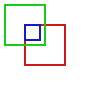```nofill()
strokewidth(2)

rectmode(CORNER)  # default, red
stroke(0.8, 0.1, 0.1)
rect(25, 25, 40, 40)

rectmode(CENTER)  # green
stroke(0.1, 0.8, 0.1)
rect(25, 25, 40, 40)

rectmode(CORNERS)  # blue
stroke(0.1, 0.1, 0.8)
rect(25, 25, 40, 40)
```
`ellipsemode`(mode=None)

Change the way ellipses are specified. Each mode alters the parameters necessary to draw an ellipse using the `ellipse()` function.

It works exactly the same as the `rectmode()` command.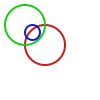```nofill()
strokewidth(2)

ellipsemode(CORNER)  # default, red
stroke(0.8, 0.1, 0.1)
ellipse(25, 25, 40, 40)

ellipsemode(CENTER)  # green
stroke(0.1, 0.8, 0.1)
ellipse(25, 25, 40, 40)

ellipsemode(CORNERS)  # blue
stroke(0.1, 0.1, 0.8)
ellipse(25, 25, 40, 40)
```

## Bézier paths¶

`beginpath`(x=None, y=None)

Begin drawing a Bézier path.

After calling beginpath(), a series of other path commands usually follows, such as moveto(), lineto(), or curveto(). Finally, the endpath() command draws the path on the screen.

If x and y are not specified, this command should be followed by a `moveto()` call.

Parameters
• x (float or None) – x-coordinate of the starting point

• y (float or None) – y-coordinate of the starting point

`moveto`(x, y)

Move the Bézier “pen” to the specified point without drawing. Can only be called between beginpath() and endpath().

Parameters
• x (float) – x-coordinate of the point to move to

• y (float) – y-coordinate of the point to move to

`lineto`(x, y)

Draw a line from the pen’s current point. Can only be called between beginpath() and endpath().

Parameters
• x (float) – x-coordinate of the point to draw to

• y (float) – y-coordinate of the point to draw to

`curveto`(x1, y1, x2, y2, x3, y3)

Draws a curve between the current point in the path and a new destination point. Can only be called between beginpath() and endpath().

The last two parameters are the coordinates of the destination point. The first 4 parameters are the coordinates of the two control points, which define the edge and slant of the curve.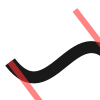```x, y = 10, 62     # Start curve point
x1, y1 = 50, 115  # Left control point
x2, y2 = 75, 10   # Right control point
x3, y3 = 115, 62  # End curve point

# Only strokes
autoclosepath(False)
nofill()

# Draw the curve
strokewidth(12)
stroke(0.1)
beginpath()
moveto(x, y)
curveto(x1, y1, x2, y2, x3, y3)
endpath()

# To show where the control points are,
# we draw helper lines
strokewidth(6)
stroke(1, 0.2, 0.2, 0.6)
# The first control point starts at the
# x, y position
line(x, y, x1, y1)
# And the second control point is the
# end curve point
line(x2, y2, x3, y3)
```
`arc`(x, y, radius, angle1, angle2)
`closepath`()

Close the path; in case the current point is not the path’s starting point, a line will be drawn between them.

`endpath`(draw=True)

The endpath() command is the companion command to beginpath(). When endpath() is called, the path defined between beginpath() and endpath() is drawn. Optionally, when endpath(draw=False) is called, the path is not drawn but can be assigned to a variable and drawn to the screen at a later time with the drawpath() command.

`drawpath`(path)

Draws a path on the screen. A path is a series of lines and curves defined between beginpath() and endpath(). Normally, endpath() draws the path to the screen, unless when calling endpath(draw=False). The path can then be assigned to a variable, and this variable used as a parameter for drawpath().

Note: if you have one path that you want to draw multiple times with drawpath(), for example each with its own rotation and position, you need to supply a copy: drawpath(path.copy())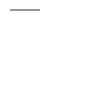```stroke(0.2)
beginpath(10, 10)
lineto(40, 10)
p = endpath(draw=False)
drawpath(p)
```
`autoclosepath`(close=True)

Defines whether paths are automatically closed by connecting the last and first points with a line. It takes a single parameter of True or False. All shapes created with beginpath() following this command will adhere to the setting.

`findpath`(points, curvature=1.0)

Constructs a fluid path from a list of coordinates. Each element in the list is a 2-tuple defining the x-coordinate and the y-coordinate. If the curve has more than three points, the curvature parameter offers some control on how separate segments are stitched together: from straight lines (0.0) to smooth curves (1.0).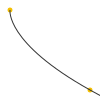```points = [(10, 10), (90, 90), (350, 200)]
ellipsemode(CENTER)
for x, y in points:
ellipse(x, y, 6, 6)

nofill()
stroke(0.2)
autoclosepath(False)
path = findpath(points)
drawpath(path)
```

## Images¶

`image`(path, x=0, y=0, width=None, height=None, alpha=1.0, data=None, draw=True)

Place a bitmap image on the canvas.

Parameters
• path (filename) – location of the image on disk

• x (float) – x-coordinate of the top left corner

• y (float) – y-coordinate of the top left corner

• width (float or None) – image width (leave blank to use its original width)

• height (float or None) – image height (leave blank to use its original height)

• alpha (float) – opacity

• data (binary data) – image data to load. Use this instead of `path` if you want to load an image from memory or have another source (e.g. using the web library)

• draw (bool) – whether to place the image immediately on the canvas or not

## Clipping paths¶

`beginclip`(path)

The beginclip() and endclip() commands define a clipping mask. The supplied parameter defines the path to be used as a clipping mask.

All basic shapes and path commands return paths that can be used with beginclip() - setting the `draw` parameter of a shape command will simply return the path without actually drawing the shape. Any shapes, paths, texts and images between beginclip() and endclip() are clipped: any part that falls outside the clipping mask path is not drawn.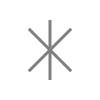```p = ellipse(20, 20, 60, 60, draw=False)
beginclip(p)
stroke(0.5)
strokewidth(3)
line(20, 20, 80, 80)
line(20, 80, 80, 20)
line(50, 20, 50, 80)
endclip()
```
`endclip`()

Used along with `beginclip()`.

## Transforms¶

`transform`(mode=None)

The mode parameter sets the registration point – the offset for rotate(), scale() and skew() commands. By default, primitives, text, and images rotate around their own centerpoints. But if you call transform() with CORNER as its mode parameter, transformations will be applied relative to the canvas ‘origin point’ rather than being relative to the objects’ centerpoint origins.

Each command example below shows how the transform mode affects the result.

Parameters

mode (CORNER or CENTER) – the mode to base new transformations on

`translate`(xt, yt)

Specifies the amount to move a subsequent shape, path, text, image on the screen. Once called, all commands following translate() are repositioned, which makes translate() useful for positioning whole compositions of multiple elements.

Parameters
• xt – horizontal offset

• yt – vertical offset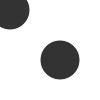```fill(0.2)
oval(-10, -10, 40, 40)
translate(50, 50)
oval(-10, -10, 40, 40)
```
`rotate`(degrees=0, radians=0)

Rotates all subsequent drawing commands. The default unit is degrees; radians can be used with `rotate(radians=PI)`. Like other transform operations, the rotate() command works incrementally: if you call rotate(30), and later on call rotate(60), all commands following that second rotate() will be rotated 90° (30+60).

param degrees

angle in degrees

param radians

angle in radians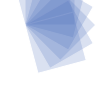```fill('#4a69bd', 0.2)
translate(25, 25)
for i in range(7):
rotate(15)
rect(0, 0, 50, 50)
```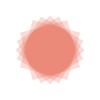```fill('#e55039', 0.2)
transform(CENTER)
for i in range(5):
rotate(15)
rect(25, 25, 50, 50)
```
`scale`(x=1, y=None)

Increases, decreases, or streches the size of all subsequent drawing commands. The first parameter sets the horizontal scale and the optional second parameter the vertical scale. You can also call scale() with a single parameter that sets both the horizontal and vertical scale. Scale values are specified as floating-point (decimal) numbers with 1.0 corresponding to 100%.

The scale() command works incrementally: if you call scale(0.5), and later on call scale(0.2), all subsequent drawing commands will be sized to 10% (0.2 of 0.5).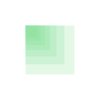```fill('#78e08f', 0.2)
translate(25,25)
for i in range(7):
rect(0, 0, 50, 50)
scale(.8)
```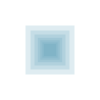```fill('#60a3bc', 0.2)
transform(CENTER)
for i in range(7):
rect(25, 25, 50, 50)
scale(.8)
```
`skew`(x=1, y=0)

Slants the direction of all subsequent drawing commands. The first parameter sets the horizontal skew. The second parameter is optional and sets the vertical skew.

The skew() command works incrementally: if you call skew(10), and later on call skew(20), all subsequent drawing commands will be skewed by 30° (10+20).```fill('#82ccdd', 0.2)
translate(5, 25)
for i in range(7):
rect(0, 0, 50, 50)
skew(.2, 0)
```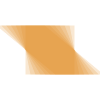```fill('#e58e26', 0.2)
transform(CENTER)
for i in range(7):
rect(25, 25, 50, 50)
skew(.2, 0)
```
`push`()

The push() function, along with its companion pop(), allows for “saving” a transform state. All transformations, such as rotate() and skew(), defined between a push() and pop() call last only until pop() is called.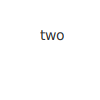```fill(0.2)
fontsize(14)
rotate(90)
text("one", 40, 80)

push()
rotate(-90)
text("two", 40, 40)
pop()

text("three", 50, 80)
```
`pop`()

The pop() function is meant to be used after push(). It “loads” the transform state that was set before the call to push().

`reset`()

Resets the transform state to its default values.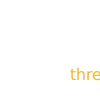```rotate(90)
text("one", 30, 80)
text("two", 45, 80)

reset()
text("three", 70, 80)
```

## Colors¶

Colors can be specified in a few ways:

• grayscale: `(value)`

• grayscale with alpha: `(value, alpha)`

• RGB: `(red, green, blue)`

• RGBA: `(red, green, blue, alpha)`

• hex: `('#FFFFFF')`

• hex with alpha: `('#FFFFFFFF')`

`background`(*args)

Set the background color.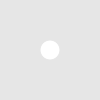```background(0.9)
fill(1)
circle(40, 40, 20)
```
`colormode`(mode=None, crange=None)

Set the current color mode (can be RGB or HSB) and eventually the color range.

Parameters
• mode (RGB or HSB) – Color mode to use

• crange – Maximum value for the new color range to use. See `colorrange`_.

Returns

Current color mode (if called without arguments)

`colorrange`(crange=1.0)

Set the numeric range for color values. By default colors range from 0.0 - 1.0, and this command can set this to a different range. For example, a scale of 0 to 255 can be set with `colorrange(255)`.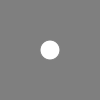```colorrange(255)
background(127)
fill(255)
circle(40, 40, 20)
```
`fill`(color)

Sets a fill color, applying it to new paths.

Parameters

color – color in supported format (see above)

`stroke`(color)

Set a stroke color, applying it to new paths.

Parameters

color – color in supported format (see above)

`nofill`()

Stop applying fills to new paths.

`nostroke`()

Stop applying strokes to new paths.

`strokewidth`(w=None)
Parameters

w – Stroke width

Returns

Current width (if no width was specified)

`color`(*args)
Parameters

args – color in a supported format

Returns

Color object

## Text¶

`text`(txt, x, y, width=None, height=1000000, outline=False, draw=True)

Draws a string of text according to current font settings.

Parameters
• txt – Text to output

• x – x-coordinate of the top left corner

• y – y-coordinate of the top left corner

• width – text box width. When set, text will wrap to the next line if it would exceed this width. If unset, there will be no line breaks.

• height – text box height

• outline (bool) – whether to draw as an outline.

• draw (bool) – if False, the object won’t be immediately drawn to canvas.

Returns

BezierPath object representing the text```# when using text(), the origin point
# is on the text baseline
ellipsemode(CENTER)
circle(12, 65, 10, fill='#ff0033')
# place the text box
font("Inconsolata", 50)
text("Bot", 12, 65)
```

`font`(fontpath=None, fontsize=None)

Set the font to be used in new text instances.

Accepts a system font name, e.g. “Inconsolata Bold”. A full list of your system’s font names can be viewed with the pango-list command in a terminal.

Parameters
• fontpath – font name

• fontsize – font size in points

Returns

current font name (if `fontpath` was not set)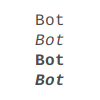```fill(0.3)
fontsize(16)

font("Liberation Mono")
text("Bot", 35, 25)
font("Liberation Mono Italic")
text("Bot", 35, 45)
font("Liberation Mono Bold")
text("Bot", 35, 65)
font("Liberation Mono Bold Italic")
text("Bot", 35, 85)
```

Variable fonts are supported. You can specify the value for an axis using keyword arguments with the `var_` prefix: to set the `wdth` axis to `100`, use `var_wdth=100`.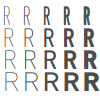```fill(0.3)
fontsize(30)

for x, y in grid(5, 4, 20, 22):
font("Inconsolata", var_wdth=y+50, var_wght=x*12)
text("R", 3+x, 25+y)
```

Note that for the above example to work, you need to install the variable version of Inconsolata.

`fontsize`(fontsize=None)

Set or return size of current font.

Parameters

fontsize – Font size in points (pt)

Returns

Font size in points (if `fontsize` was not specified)

`textpath`(txt, x, y, width=None, height=1000000, draw=False)

Generates an outlined path of the input text.

Parameters
• txt – Text to output

• x – x-coordinate of the top left corner

• y – y-coordinate of the top left corner

• width – text width

• height – text height

• draw – Set to False to inhibit immediate drawing (defaults to False)

Returns

Path object representing the text.

`textmetrics`(txt, width=None, height=None)
Returns

the width and height of a string of text as a tuple (according to current font settings).

`textwidth`(txt, width=None)
Parameters

text – the text to test for its dimensions

Returns

the width of a string of text according to the current font settings

`textheight`(txt, width=None)
Parameters

text – the text to test for its dimensions

Returns

the height of a string of text according to the current font settings

`lineheight`(height=None)

Set the space between lines of text.

Parameters

height – line height

`align`(align=LEFT)

Set the way lines of text align with each other.

Parameters

align (LEFT, CENTER or RIGHT) – Text alignment rule

`fontoptions`(hintstyle=None, hintmetrics=None, subpixelorder=None, antialias=None)

Not implemented.

## Dynamic variables¶

`var`(name, type, default=None, min=0, max=255, value=None, step=None, steps=256.0)

Create a live variable.

Parameters
• name – Variable name

• type (NUMBER, TEXT, BOOLEAN or BUTTON) – Variable type

• default – Default value

• min – Minimum value (NUMBER only)

• max – Maximum value (NUMBER only)

• value – Initial value (if not defined, use `default`)

• step – Step length for the variables GUI (use this or `steps`, not both)

• steps – Number of steps in the variables GUI (use this or `step`, not both)

## Utility functions¶

`random`(v1=None, v2=None)

Returns a random number that can be assigned to a variable or a parameter. When no parameters are supplied, returns a floating-point (decimal) number between 0.0 and 1.0 (including 0.0 and 1.0). When one parameter is supplied, returns a number between 0 and this parameter. When two parameters are supplied, returns a number between the first and the second parameter.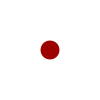```r = random() # returns a float between 0 and 1
r = random(2.5) # returns a float between 0 and 2.5
r = random(-1.0, 1.0) # returns a float between -1.0 and 1.0
r = random(5) # returns an int between 0 and 5
r = random(1, 10) # returns an int between 1 and 10

# sets the fill to anything from
# black (0.0,0,0) to red (1.0,0,0)
fill(random(), 0, 0)
circle(40, 40, 20)

# Note: new random values are returned each time the script runs.
# The variation can be locked by supplying a custom random seed:

from random import seed
seed(0)
```
`grid`(cols, rows, colSize=1, rowSize=1, shuffled=False)

The grid() command returns an iteratable object, something that can be traversed in a for-loop (like the range() command for example).

The grid() is a complex but powerful command. The first two parameters define the number of columns and rows in the grid. The next two parameters are optional, and set the width and height of one cell in the grid. In each iteration of a for-loop, the offset for the current column and row is returned.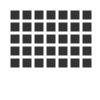```fill(0.2)
for x, y in grid(7, 5, 12, 12):
rect(10+x, 10+y, 10, 10)
```
`files`(path='*')

Retrieves all files from a given path and returns their names as a list. Wildcards can be used to specify which files to pick, e.g. ```f = files('*.gif')```

Parameters

path – wildcard to use in file list

`autotext`(sourceFile)

Generates mock philosophy based on a context-free grammar.

Parameters

sourcefile – file path to use as source

Returns

the generated text

`snapshot`(filename=None, surface=None, defer=None, autonumber=False)

Save the contents of current surface into a file or cairo surface/context.

Parameters
• filename – File name to output to. The file type will be deduced from the extension.

• surface – If specified will output snapshot to the supplied cairo surface.

• defer (boolean) – Decides whether the action needs to happen now or can happen later. When set to False, it ensures that a file is written before returning, but can hamper performance. Usually you won’t want to do this. For files defer defaults to True, and for Surfaces to False, this means writing files won’t stop execution, while the surface will be ready when snapshot returns. The drawqueue will have to stop and render everything up until this point.

• autonumber (boolean) – If true then a number will be appended to the filename.

## Core¶

`ximport`(libName)

Import Nodebox libraries.

The libraries get access to the _ctx context object, which provides them with the Shoebot API.

Parameters

libName – Library name to import

`size`(w=None, h=None)

Sets the size of the canvas, and creates a Cairo surface and context. Only the first call will have any effect.

`speed`(framerate)

Set the framerate for animations.

Parameters

framerate – Frames per second

Returns

Current framerate

`run`(inputcode, iterations=None, run_forever=False, frame_limiter=False)

Executes the contents of a Shoebot script in the current surface’s context.

## Classes¶

class `BezierPath`
class `Text`